Author: Oscar Cronquist Article last updated on October 31, 2018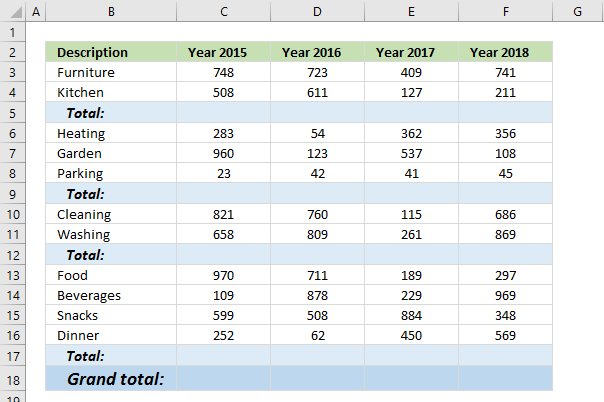Is it possible to quickly select all empty cells and then sum cells above to next empty cell?

Can I have a formula in grand total (row 18) that only sums all the totals above?

### Instructions

Select all values and the blank total cells.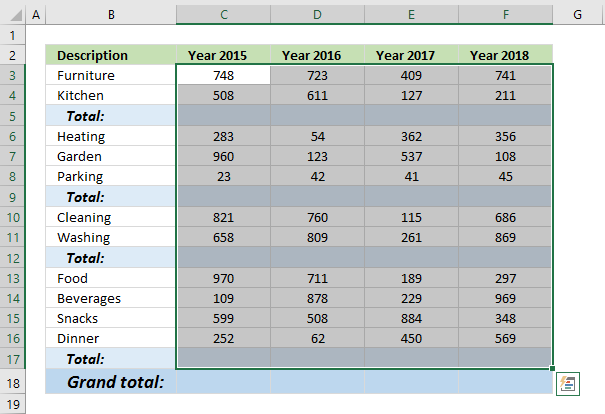Press F5 or go to tab "Home" on the ribbon, click on "Find & Select" button and then click on "Go To.."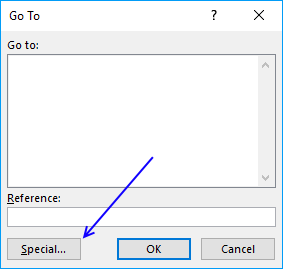Click on "Special..." button.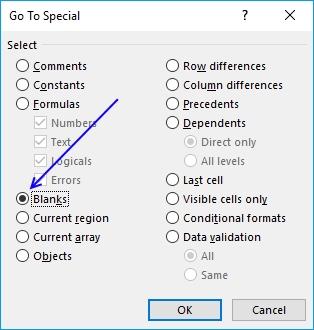Click on "Blanks" and then on "OK" button.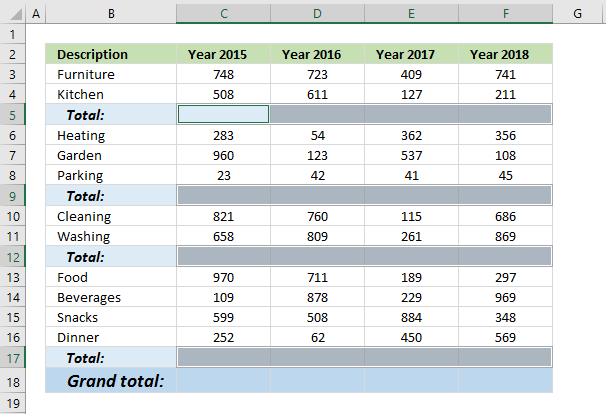Go to tab "Home" on the ribbon and click on "AutoSum" button.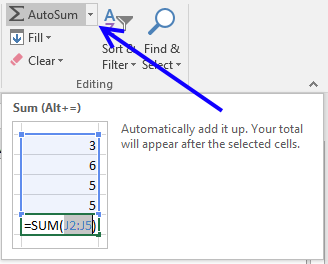All empty cells now have a SUM formula that adds all above values to the next SUM formula.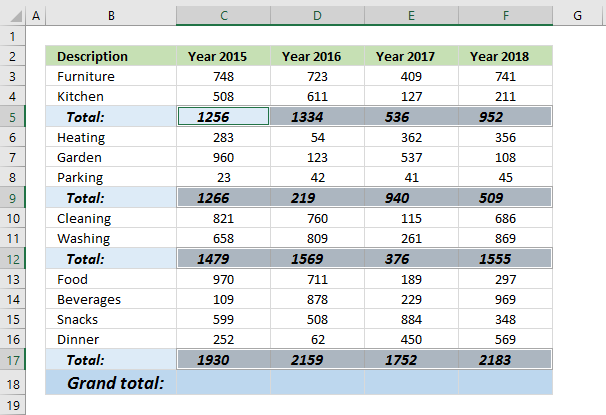Select cell C18 and type this formula:

=SUMPRODUCT(ISFORMULA(C3:C17)*C3:C17)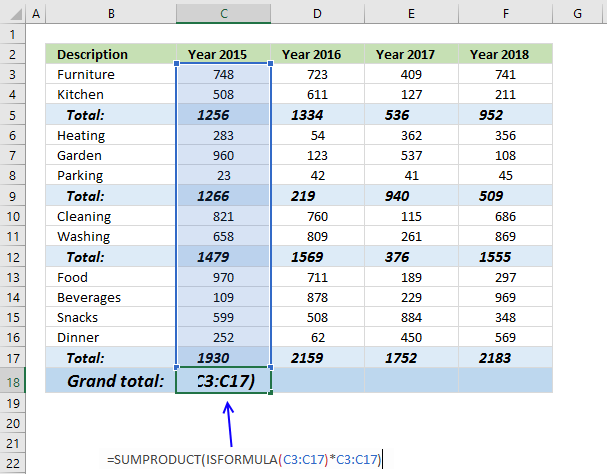Press Enter. Copy cell C18 and paste to cell range D18:F18.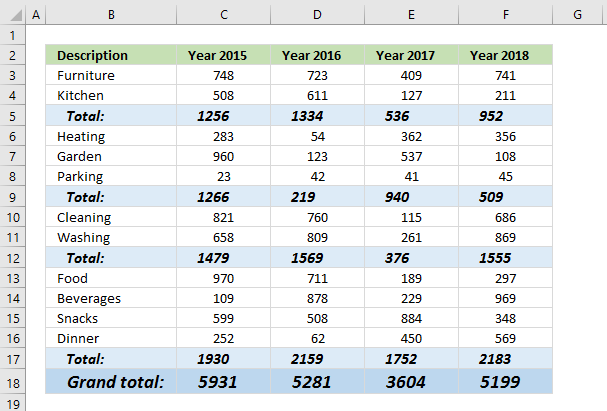### Explaining formula in cell C18

ISFORMULA(C3:C17) checks if a cell in cell range C3:C17 has a formula. It returns TRUE or FALSE.

ISFORMULA(C3:C17) returns this array: {FALSE; FALSE; TRUE; FALSE; FALSE; FALSE; TRUE; FALSE; FALSE; TRUE; FALSE; FALSE; FALSE; FALSE; TRUE}

The picture below shows this array in column D.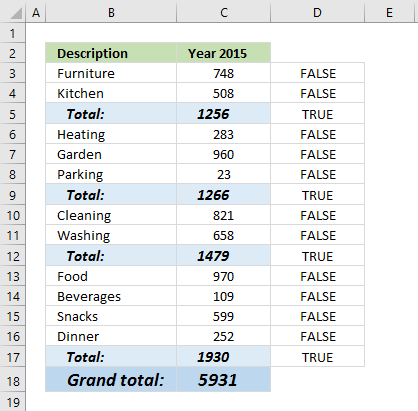The array shows that there is a formula in C5, C9, C12 and C17.

ISFORMULA(C3:C17)*C3:C17 multiplies the boolean values with their corresponding values in column C, shown in column E below.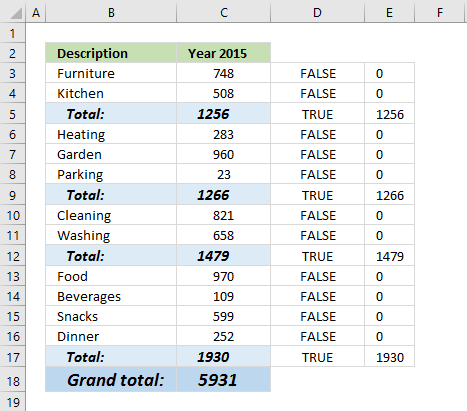The SUMPRODUCT function then sums all values in the array. Why not use the SUM function? You need to enter it as an array formula if you use the SUM function.

### Download excel *.xlsx file

Find empty cells and sum cells above.xlsx